Publicité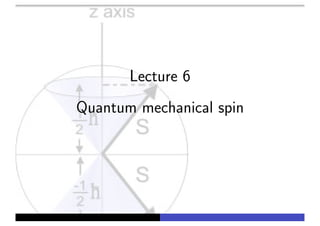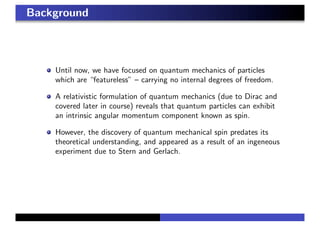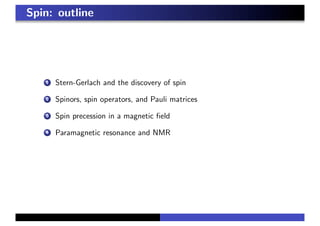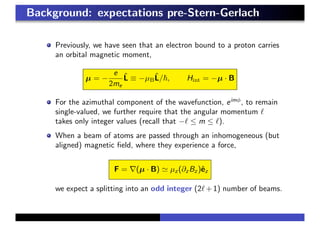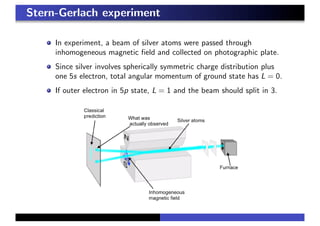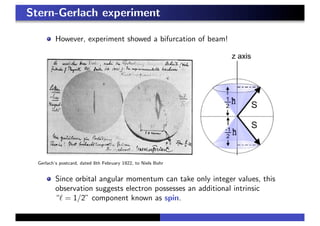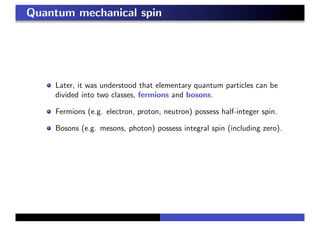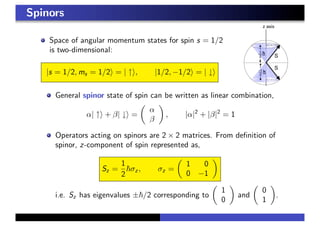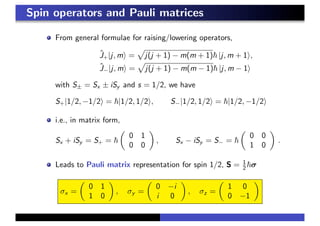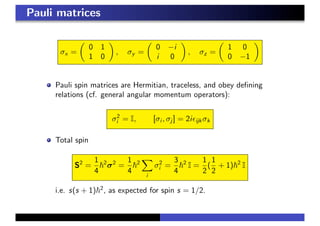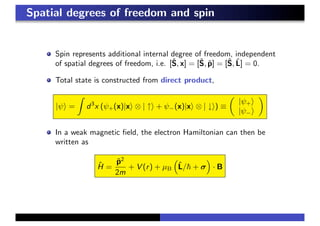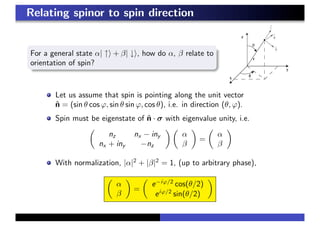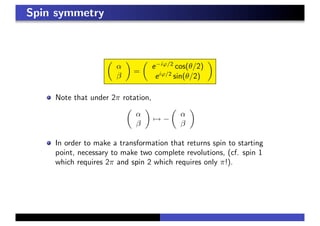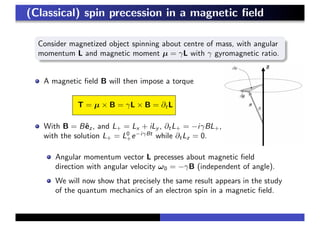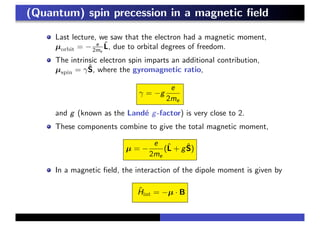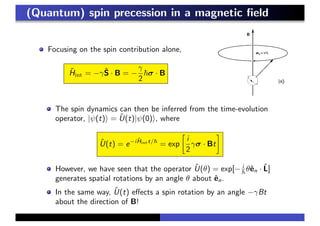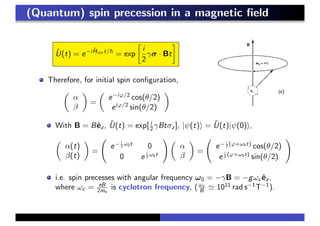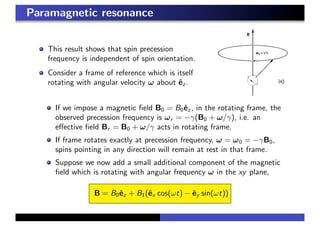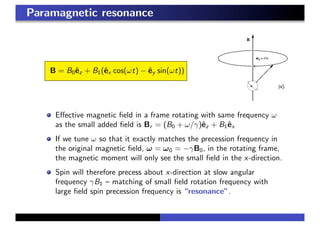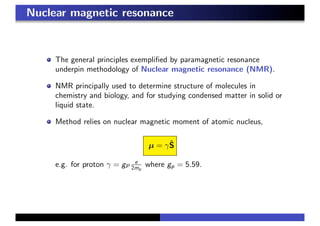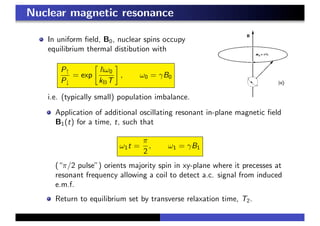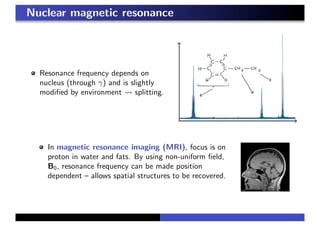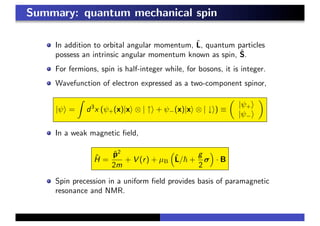1 sur 23
Publicité

### Quantum mechanical spin

1. Lecture 6 Quantum mechanical spin
2. Background Until now, we have focused on quantum mechanics of particles which are “featureless” – carrying no internal degrees of freedom. A relativistic formulation of quantum mechanics (due to Dirac and covered later in course) reveals that quantum particles can exhibit an intrinsic angular momentum component known as spin. However, the discovery of quantum mechanical spin predates its theoretical understanding, and appeared as a result of an ingeneous experiment due to Stern and Gerlach.
3. Spin: outline 1 Stern-Gerlach and the discovery of spin 2 Spinors, spin operators, and Pauli matrices 3 Spin precession in a magnetic field 4 Paramagnetic resonance and NMR
4. Background: expectations pre-Stern-Gerlach Previously, we have seen that an electron bound to a proton carries an orbital magnetic moment, µ = − e 2me L̂ ≡ −µBL̂/!, Hint = −µ · B For the azimuthal component of the wavefunction, eimφ , to remain single-valued, we further require that the angular momentum ! takes only integer values (recall that −! ≤ m ≤ !). When a beam of atoms are passed through an inhomogeneous (but aligned) magnetic field, where they experience a force, F = ∇(µ · B) % µz (∂z Bz )êz we expect a splitting into an odd integer (2!+1) number of beams.
5. Stern-Gerlach experiment In experiment, a beam of silver atoms were passed through inhomogeneous magnetic field and collected on photographic plate. Since silver involves spherically symmetric charge distribution plus one 5s electron, total angular momentum of ground state has L = 0. If outer electron in 5p state, L = 1 and the beam should split in 3.
6. Stern-Gerlach experiment However, experiment showed a bifurcation of beam! Gerlach’s postcard, dated 8th February 1922, to Niels Bohr Since orbital angular momentum can take only integer values, this observation suggests electron possesses an additional intrinsic “! = 1/2” component known as spin.
7. Quantum mechanical spin Later, it was understood that elementary quantum particles can be divided into two classes, fermions and bosons. Fermions (e.g. electron, proton, neutron) possess half-integer spin. Bosons (e.g. mesons, photon) possess integral spin (including zero).
8. Spinors Space of angular momentum states for spin s = 1/2 is two-dimensional: |s = 1/2, ms = 1/2& = | ↑&, |1/2, −1/2& = | ↓& General spinor state of spin can be written as linear combination, α| ↑& + β| ↓& = ! α β " , |α|2 + |β|2 = 1 Operators acting on spinors are 2 × 2 matrices. From definition of spinor, z-component of spin represented as, Sz = 1 2 !σz , σz = ! 1 0 0 −1 " i.e. Sz has eigenvalues ±!/2 corresponding to ! 1 0 " and ! 0 1 " .
9. Spin operators and Pauli matrices From general formulae for raising/lowering operators, Ĵ+|j, m& = # j(j + 1) − m(m + 1)! |j, m + 1&, Ĵ−|j, m& = # j(j + 1) − m(m − 1)! |j, m − 1& with S± = Sx ± iSy and s = 1/2, we have S+|1/2, −1/2& = !|1/2, 1/2&, S−|1/2, 1/2& = !|1/2, −1/2& i.e., in matrix form, Sx + iSy = S+ = ! ! 0 1 0 0 " , Sx − iSy = S− = ! ! 0 0 1 0 " . Leads to Pauli matrix representation for spin 1/2, S = 1 2 !σ σx = ! 0 1 1 0 " , σy = ! 0 −i i 0 " , σz = ! 1 0 0 −1 "
10. Pauli matrices σx = ! 0 1 1 0 " , σy = ! 0 −i i 0 " , σz = ! 1 0 0 −1 " Pauli spin matrices are Hermitian, traceless, and obey defining relations (cf. general angular momentum operators): σ2 i = I, [σi , σj ] = 2i&ijk σk Total spin S2 = 1 4 !2 σ2 = 1 4 !2 \$ i σ2 i = 3 4 !2 I = 1 2 ( 1 2 + 1)!2 I i.e. s(s + 1)!2 , as expected for spin s = 1/2.
11. Spatial degrees of freedom and spin Spin represents additional internal degree of freedom, independent of spatial degrees of freedom, i.e. [Ŝ, x] = [Ŝ, p̂] = [Ŝ, L̂] = 0. Total state is constructed from direct product, |ψ& = % d3 x (ψ+(x)|x& ⊗ | ↑& + ψ−(x)|x& ⊗ | ↓&) ≡ ! |ψ+& |ψ−& " In a weak magnetic field, the electron Hamiltonian can then be written as Ĥ = p̂2 2m + V (r) + µB & L̂/! + σ ' · B
12. Relating spinor to spin direction For a general state α| ↑& + β| ↓&, how do α, β relate to orientation of spin? Let us assume that spin is pointing along the unit vector n̂ = (sin θ cos ϕ, sin θ sin ϕ, cos θ), i.e. in direction (θ, ϕ). Spin must be eigenstate of n̂ · σ with eigenvalue unity, i.e. ! nz nx − iny nx + iny −nz " ! α β " = ! α β " With normalization, |α|2 + |β|2 = 1, (up to arbitrary phase), ! α β " = ! e−iϕ/2 cos(θ/2) eiϕ/2 sin(θ/2) "
13. Spin symmetry ! α β " = ! e−iϕ/2 cos(θ/2) eiϕ/2 sin(θ/2) " Note that under 2π rotation, ! α β " +→ − ! α β " In order to make a transformation that returns spin to starting point, necessary to make two complete revolutions, (cf. spin 1 which requires 2π and spin 2 which requires only π!).
14. (Classical) spin precession in a magnetic field Consider magnetized object spinning about centre of mass, with angular momentum L and magnetic moment µ = γL with γ gyromagnetic ratio. A magnetic field B will then impose a torque T = µ × B = γL × B = ∂tL With B = Bêz , and L+ = Lx + iLy , ∂tL+ = −iγBL+, with the solution L+ = L0 +e−iγBt while ∂tLz = 0. Angular momentum vector L precesses about magnetic field direction with angular velocity ω0 = −γB (independent of angle). We will now show that precisely the same result appears in the study of the quantum mechanics of an electron spin in a magnetic field.
15. (Quantum) spin precession in a magnetic field Last lecture, we saw that the electron had a magnetic moment, µorbit = − e 2me L̂, due to orbital degrees of freedom. The intrinsic electron spin imparts an additional contribution, µspin = γŜ, where the gyromagnetic ratio, γ = −g e 2me and g (known as the Landé g-factor) is very close to 2. These components combine to give the total magnetic moment, µ = − e 2me (L̂ + gŜ) In a magnetic field, the interaction of the dipole moment is given by Ĥint = −µ · B
16. (Quantum) spin precession in a magnetic field Focusing on the spin contribution alone, Ĥint = −γŜ · B = − γ 2 !σ · B The spin dynamics can then be inferred from the time-evolution operator, |ψ(t)& = Û(t)|ψ(0)&, where Û(t) = e−iĤintt/! = exp ( i 2 γσ · Bt ) However, we have seen that the operator Û(θ) = exp[− i ! θên · L̂] generates spatial rotations by an angle θ about ên. In the same way, Û(t) effects a spin rotation by an angle −γBt about the direction of B!
17. (Quantum) spin precession in a magnetic field Û(t) = e−iĤintt/! = exp ( i 2 γσ · Bt ) Therefore, for initial spin configuration, ! α β " = ! e−iϕ/2 cos(θ/2) eiϕ/2 sin(θ/2) " With B = Bêz , Û(t) = exp[ i 2 γBtσz ], |ψ(t)& = Û(t)|ψ(0)&, ! α(t) β(t) " = * e− i 2 ω0t 0 0 e i 2 ω0t + ! α β " = * e− i 2 (ϕ+ω0t) cos(θ/2) e i 2 (ϕ+ω0t) sin(θ/2) + i.e. spin precesses with angular frequency ω0 = −γB = −gωc êz , where ωc = eB 2me is cyclotron frequency, (ωc B % 1011 rad s−1 T−1 ).
18. Paramagnetic resonance This result shows that spin precession frequency is independent of spin orientation. Consider a frame of reference which is itself rotating with angular velocity ω about êz . If we impose a magnetic field B0 = B0êz , in the rotating frame, the observed precession frequency is ωr = −γ(B0 + ω/γ), i.e. an effective field Br = B0 + ω/γ acts in rotating frame. If frame rotates exactly at precession frequency, ω = ω0 = −γB0, spins pointing in any direction will remain at rest in that frame. Suppose we now add a small additional component of the magnetic field which is rotating with angular frequency ω in the xy plane, B = B0êz + B1(êx cos(ωt) − êy sin(ωt))
19. Paramagnetic resonance B = B0êz + B1(êx cos(ωt) − êy sin(ωt)) Effective magnetic field in a frame rotating with same frequency ω as the small added field is Br = (B0 + ω/γ)êz + B1êx If we tune ω so that it exactly matches the precession frequency in the original magnetic field, ω = ω0 = −γB0, in the rotating frame, the magnetic moment will only see the small field in the x-direction. Spin will therefore precess about x-direction at slow angular frequency γB1 – matching of small field rotation frequency with large field spin precession frequency is “resonance”.
20. Nuclear magnetic resonance The general principles exemplified by paramagnetic resonance underpin methodology of Nuclear magnetic resonance (NMR). NMR principally used to determine structure of molecules in chemistry and biology, and for studying condensed matter in solid or liquid state. Method relies on nuclear magnetic moment of atomic nucleus, µ = γŜ e.g. for proton γ = gP e 2mp where gp = 5.59.
21. Nuclear magnetic resonance In uniform field, B0, nuclear spins occupy equilibrium thermal distibution with P↑ P↓ = exp ( !ω0 kBT ) , ω0 = γB0 i.e. (typically small) population imbalance. Application of additional oscillating resonant in-plane magnetic field B1(t) for a time, t, such that ω1t = π 2 , ω1 = γB1 (“π/2 pulse”) orients majority spin in xy-plane where it precesses at resonant frequency allowing a coil to detect a.c. signal from induced e.m.f. Return to equilibrium set by transverse relaxation time, T2.
22. Nuclear magnetic resonance Resonance frequency depends on nucleus (through γ) and is slightly modified by environment ! splitting. In magnetic resonance imaging (MRI), focus is on proton in water and fats. By using non-uniform field, B0, resonance frequency can be made position dependent – allows spatial structures to be recovered.
23. Summary: quantum mechanical spin In addition to orbital angular momentum, L̂, quantum particles possess an intrinsic angular momentum known as spin, Ŝ. For fermions, spin is half-integer while, for bosons, it is integer. Wavefunction of electron expressed as a two-component spinor, |ψ& = % d3 x (ψ+(x)|x& ⊗ | ↑& + ψ−(x)|x& ⊗ | ↓&) ≡ ! |ψ+& |ψ−& " In a weak magnetic field, Ĥ = p̂2 2m + V (r) + µB & L̂/! + g 2 σ ' · B Spin precession in a uniform field provides basis of paramagnetic resonance and NMR.
Publicité